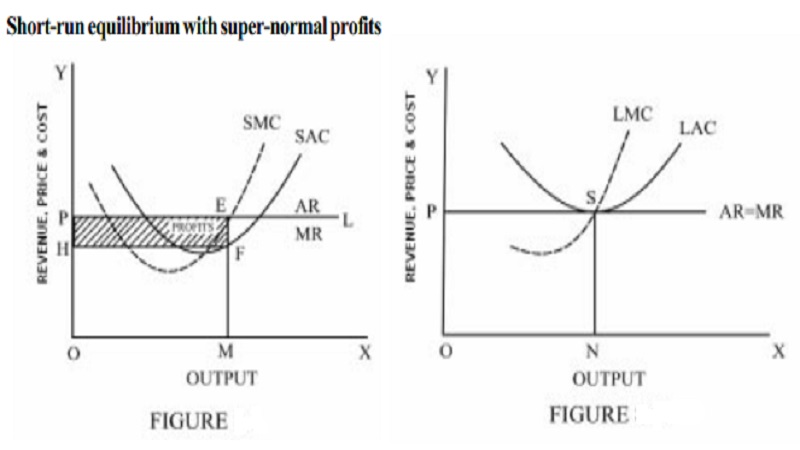Home | | Economics | | Economics | Nature of Revenue curves

# Nature of Revenue curvesNature of Revenue curves: 1.Short run equilibrium price and output determination under per-fect competition. 2.Long run equilibrium, price and output determination. Advantages of perfect competition

Nature of Revenue curves

Under perfect competition, the market price is determined by the market forces namely the demand for and the supply of the products. Hence there is uniform price in the market and all the units of the output are sold at the same price. As a result the average revenue is perfectly elastic. The average revenue curve is horizontally parallel to X-axis. Since the Average Revenue is constant, Marginal Revenue is also constant and coincides with Average Revenue. AR curve of a firm represents the demand curve for the product produced by that firm.

## Short run equilibrium price and output determination under per-fect competition

Since a firm in the perfectly competitive market is a price-taker, it has to adjust its level of output to maximise its profit. The aim of any producer is to maximise his profit.

The short run is a period in which the number and plant size of the firms are fixed. In this period, the firm can produce more only by increasing the variable inputs.

As the entry of new firms or exit of the existing firms are not possible in the short-run, the firm in the perfectly competitive market can either earn super-normal profit or normal profit or incur loss in the short period.

## Super-normal Profit

When the average revenue of the firm is greater than its average cost, the firm is earning super-normal profit.

In figure , output is measured along the x-axis and price, revenue and cost along the y-axis. OP is the prevailing price in the market. PL is the demand curve or average and the marginal revenue curve. SAC and SMC are the short run average and marginal cost curves. The firm is in equilibrium at point 'E' where MR = MC and MC curve cuts MR curve from below at the point of equilibrium. Therefore the firm will be producing OM level of output. At the OM level of output ME is the AR and MF is the average cost. The profit per unit of output is EF (the difference between ME and MF). The total profits earned by the firm will be equal to EF (profit per unit) multiplied by OM or HF (total output). Thus the total profits will be equal to the area HFEP. HFEP is the supernormal profits earned by the firm.

## Long run equilibrium, price and output determination

In the long run, all factors are variable. The firms can increase their output by increasing the number and plant size of the firms. Moreover, new firms can enter the industry and the existing firms can leave the industry. As a result, all the existing firms will earn only normal profit in the long run.

If the existing firms earn supernormal profit, the new firms will enter the industry to compete with the existing firms. As a result, the output produced will increase. When the total output increases, the demand for factors of production will increase leading to increase in prices of the factors. This will result in increase in average cost.

On the other side, when the output produced increases, the supply of the product increases. The demand remaining the same, when the supply of the product increases, the price of the product comes down. Hence the average revenue will come down. A fall in average revenue and the rise in average cost will continue till both become equal. (AR = AC). Thus, all the perfectly competitive firms will earn normal profit in the long run.

Figure  represents long run equilibrium of firm under perfect competition. The firm is in equilibrium at point S where LMC = MR = AR = LAC. The long run equilibrium output is ON. The firm is earning just the normal profit. The equilibrium price is OP. If the price rises above OP, the firm will earn abnormal profit, which will attract new firms into the industry. If the price is less than OP, there will be loss and the tendency will be to exit. So in the long run equilibrium, OP will be the price and marginal cost will be equal to average cost and average revenue. Thus the firm in the long run will earn only normal profit. Competitive firms are in equilibrium at the minimum point of LAC curve. Operating at the minimum point of LAC curve signifies that the firm is of optimum size i.e. producing output at the lowest possible average cost.

1.        There is consumer sovereignty in a perfect competitive market. The consumer is rational and he has perfect knowledge about the market conditions. Therefore, he will not purchase the products at a higher price.

2.        In the perfectly competitive market, the price is equal to the minimum average cost. It is beneficial to the consumer.

3.        The perfectly competitive firms are price-takers and the products are homogeneous. Therefore it is not necessary for the producers to incur expenditure on advertisement to promote sales. This reduces the wastage of resources.

In the long run, the perfectly competitive firm is functioning at the optimum level. This means that maximum economic efficiency in production is achieved. As the actual output produced by the firm is equal to the optimum output, there is no idle or unused or excess capacity.

Study Material, Lecturing Notes, Assignment, Reference, Wiki description explanation, brief detail

Related Topics